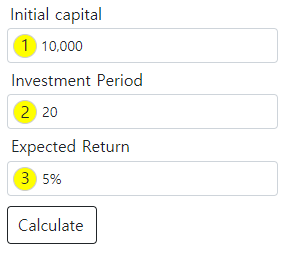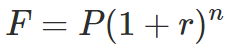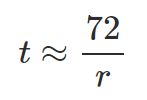# Compound Interest Calculator

Total Earned
Future Balance
# Earnings Balance Yield

## How to use

Compound Interest Calculator is a tool for calculating returns when investing in stocks or cryptocurrencies with compound interest.• Starting Amount : Enter the starting amount.
• Number of Times : Enter the investment period. Compound interest is repeated as many times as you enter it.
• Rate of Return : Enter the expected compound interest rate.
• Calculate : Outputs the result calculated with compound interest.

## What is compound interest?

Compound interest is a method of calculating the next interest by combining the principal and the previous interest when calculating the interest of the investment asset. Unlike simple interest investments, which always generate the same interest, compound interest investments continue to increase interest. So compound interest investments exponentially increase assets over time.

# Starting Balance Earnings Yield Balance
1 10000 1000 10% 11000
2 11000 1100 21% 12100
3 12100 1210 33.1% 13310

For example, assuming an initial capital of 10000 and an annual increase of 10%, interest will increase by 10% in the first year, but by 11% in the second year. Finally, in the third year, the total amount is 13310, an increase of 33.1% from initial capital. This is 3.1 percentage points larger than assuming simple interest.

## Compound interest formula

When investing with compound interest, the future value of an asset can be calculated in the following way.• F : Future value
• P : Present value
• r : Interest rate
• n : Number of compounding periods

The formula for compound interest used in this calculator follows this formula.

## Rule of 72

The Rule of 72 is a mental arithmetic method of calculating the time required to double an asset through compound interest.The method is simply to divide 72 by compound interest rate. For example, if the interest rate is 5%, the time required for an asset to double is 72/5 = 14.4 years. This is very close to the real time period of 14.207 years. This is useful in situations where you don't have a calculator.Since it is mathematically more accurate to use 69.3 instead of 72, we may use the rule of 69 or the rule of 70. However, the rule of 72 is usually used more often because 72 is divided into more natural numbers.

## Purpose of use

Most investment assets can yield compounded returns. When investing in stocks, cryptocurrencies, and FX margins, first calculate your future profit through the compound interest calculator and design your future plan.